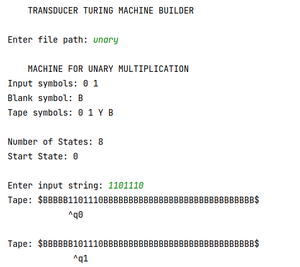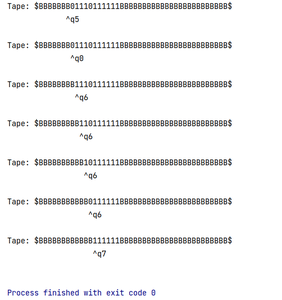# Turing Machine Construction (Transducers Turing Machine) in Java

• Difficulty Level : Medium
• Last Updated : 15 Dec, 2021

Prerequisite Turing Machine

Turing Machines can broadly be classified into two types, the Acceptors and the Transducers. Acceptor Turing Machine is an automaton used to define Turing-acceptable languages. Such a machine can be used to check whether a given string belongs to a language or not. It is defined as a 7-tuple machine.

Coming to Transducers: In general, transducers are the devices used to convert one form of signal into another. The same can be told about Turing Machine Transducers.

A transducer is a type of Turing Machine that is used to convert the given input into the output after the machine performs various read-writes. It doesn’t accept or reject an input but performs series of operations to obtain the output right in the same tape and halts when finished.

Few examples of Turing Machine transducers are:

Implementation:

Now we will be proposing a Java program that was written to simulate the construction and execution performed by turing machine transducers. There are two inputs that must be given while executing it: A .txt file to define the automaton (an example for unary multiplication machine is given after the code), and a string to be entered via the console window which will be the input on tape for the automaton to execute.

The .txt file’s path must be given as input. This was done so that the same program can be used for various types of machines instead of hard-coding the automaton. We just need to write a different txt file for generating a different automaton.

Java was chosen specifically because of the OOP structure, using which a class was defined for State, Transition, Machine, etc, to be able to encapsulate various aspects of an entity within an object. For example, a transition is defined as a class whose members are three characters – read, write and shift which store read symbol, write a symbol, and shift direction respectively, along with the index of the next state to which the machine should transition to. The same applies to a State object, which stores a list of possible outgoing transitions.

Example

## Java

 `// Java Program to Illustrate Construction of Turing Machine` `// Importing package``package` `turing_machine;` `// Importing required classes``import` `java.io.File;``import` `java.io.FileNotFoundException;``import` `java.util.ArrayList;``import` `java.util.Scanner;` `// Class 1``// Helper class``class` `Transition {` `    ``char` `read;``    ``char` `write;``    ``char` `shift;``    ``int` `nextState;` `    ``// Constructor``    ``// This divides string into specific symbols``    ``// and next state's number``    ``Transition(String s)``    ``{` `        ``read = s.charAt(``0``);``        ``write = s.charAt(``2``);``        ``shift = s.charAt(``4``);` `        ``int` `l = s.length();``        ``String substr = s.substring(``6``, l);``        ``nextState = Integer.parseInt(substr);``    ``}``}` `// Class 2``// Helper class``class` `State {` `    ``// List of transitions for a state by``    ``// creating ArrayList object of Transaction type``    ``ArrayList trs;` `    ``State(ArrayList ts) { trs = ts; }``}` `// Class 3``// Helper class``class` `Machine {` `    ``// Scanner object to read input``    ``Scanner fs;``    ``// Number of states to be read``    ``int` `stateCount;``    ``// Initialized to start state, and then to keep track``    ``// of current state in automaton``    ``int` `currState;``    ``// To halt the machine when reached, must not contain``    ``// any transitions``    ``int` `finalState;``    ``// Blank symbol defined for the machine in the input``    ``// file``    ``char` `blankSym;` `    ``// TAPE is a member of machine``    ``StringBuffer Tape = ``new` `StringBuffer();` `    ``// List of states``    ``ArrayList states = ``new` `ArrayList<>();` `    ``// Method 1``    ``void` `buildMachine(Scanner f)``    ``{``        ``this``.fs = f;` `        ``// Printing the title in the first line of input``        ``// file``        ``System.out.println(``"\n\t"` `+ readString());` `        ``// Reading the string of input symbols (space``        ``// separated)``        ``String s = readString();``        ``System.out.println(``"Input symbols: "` `+ s);` `        ``// Reading string of other tape symbols defined in``        ``// transitions``        ``s += ``" "` `+ readString();` `        ``// Reading the blank symbol from the file``        ``blankSym = readChar();``        ``System.out.println(``"Blank symbol: "` `+ blankSym);` `        ``s += ``" "` `+ blankSym;``        ``System.out.println(``"Tape symbols: "` `+ s);` `        ``// Number of states to be defined, say N``        ``stateCount = readInt();``        ``System.out.println(``"\nNumber of States: "``                           ``+ stateCount);` `        ``// Current state variable (currState) is initialized``        ``// to start-state``        ``currState = readInt();``        ``System.out.println(``"Start State: "` `+ currState);` `        ``// addState() method is called N number of times``        ``for` `(``int` `i = ``0``; i < stateCount; i++)``            ``addState(i);``    ``}` `    ``// Method 2``    ``void` `addState(``int` `ind)``    ``{``        ``// number of transitions is read for a state and``        ``// stored in trCount``        ``int` `trCount = readInt();` `        ``// state with 0 transitions is assigned to be final``        ``// state for the machine to halt``        ``if` `(trCount == ``0``)``            ``finalState = ind;``        ``ArrayList trs = ``new` `ArrayList<>();` `        ``for` `(``int` `i = ``0``; i < trCount; i++) {` `            ``// Each transition object is created and``            ``// appended to list``            ``// of transitions``            ``String s = readString();``            ``Transition tr = ``new` `Transition(s);``            ``trs.add(tr);``        ``}` `        ``// new state object is created by passing list of``        ``// transitions with the constructor``        ``State st = ``new` `State(trs);``        ``states.add(st);``    ``}` `    ``// Method 3``    ``// To read input from file object "fs" and return it``    ``String readString()``    ``{` `        ``String s = fs.next();``        ``// To ignore lines starting from '//'``        ``while` `(s.startsWith(``"//"``) || s.isEmpty())``            ``s = fs.next();``        ``return` `s;``    ``}` `    ``// Method 4``    ``// To read input from file object as string and``    ``// return the first character``    ``char` `readChar()``    ``{` `        ``String s = fs.next();``        ``while` `(s.startsWith(``"//"``) || s.isEmpty())``            ``s = fs.next();``        ``return` `s.charAt(``0``);``    ``}` `    ``// Method 5``    ``// To read input from file object and``    ``// return it's integer form``    ``int` `readInt()``    ``{``        ``String s = fs.next();``        ``while` `(s.startsWith(``"//"``) || s.isEmpty())``            ``s = fs.next();``        ``return` `Integer.parseInt(s);``    ``}` `    ``// Method 6``    ``// To perform transitions on the tape starting from``    ``// currState``    ``void` `runTuring(``int` `index) ``throws` `InterruptedException``    ``{` `        ``while` `(currState != finalState) {` `            ``// Calling makeTrans() to perform transition and``            ``// returning the index pointed by the R/W head``            ``index = makeTrans(index);``            ``if` `(index == -``1``)``                ``throw` `new` `InterruptedException(``                    ``"ERROR: Transition Not Found! Machine HALTED."``);` `            ``// Tape instance printed after each transition``            ``printTape(index);``        ``}``    ``}` `    ``int` `makeTrans(``int` `index) ``throws` `InterruptedException``    ``{``        ``if` `(Tape.charAt(index) == ``'\$'``)``            ``throw` `new` `InterruptedException(``                ``"ERROR: Head left the Tape boundary! Machine HALTED."``);` `        ``State st = states.get(currState);` `        ``// to traverse across the list of transitions to``        ``// match tape symbol with read symbol``        ``for` `(Transition tr : st.trs) {``            ``if` `(tr.read == Tape.charAt(index)) {``                ``// to write the write-symbol onto the tape``                ``Tape.replace(index, index + ``1``,``                             ``String.valueOf(tr.write));``                ``currState = tr.nextState;` `                ``switch` `(tr.shift) {``                ``case` `'R'``:``                    ``return` `index + ``1``; ``// shift right on tape``                ``case` `'L'``:``                    ``return` `index - ``1``; ``// shift left on tape``                ``default``:``                    ``return` `-``1``; ``// unknown shift symbol``                ``}``            ``}``        ``}``        ``return` `-``1``; ``// transition not found``    ``}` `    ``void` `printTape(``int` `index)``    ``{``        ``int` `interval = ``500``; ``// in milliseconds``        ``System.out.println(``"Tape: "` `+ Tape);``        ``for` `(``int` `i = ``0``; i < index; i++)``            ``System.out.print(``" "``); ``// to align` `        ``// to print the R/W head of machine pointing to``        ``// particular tape index along with current state``        ``// index``        ``System.out.println(``"      ^q"` `+ currState + ``"\n"``);``        ``try` `{``            ``// to print new instance of tape with a``            ``// particular interval``            ``Thread.sleep(interval);``        ``}``        ``catch` `(InterruptedException e) {``            ``System.out.println(e.getMessage());``        ``}``    ``}``}` `// Class 4``// Helper class``class` `FileScanner {``    ``Scanner scan = ``new` `Scanner(System.in);``    ``Scanner fileScan;``    ``String inputstr;` `    ``FileScanner() ``throws` `FileNotFoundException``    ``{``        ``// to read the input from .txt file``        ``System.out.print(``"Enter file path: "``);``        ``String path = scan.nextLine();``        ``fileScan = ``new` `Scanner(``new` `File(path));``        ``fileScan.useDelimiter(``"\n"``);``    ``}` `    ``String buildTape(String str, ``char` `blank)``    ``{``        ``// str is the input string to be added to the tape``        ``// tape defined to begin and end with '\$' symbol to``        ``// avoid indefinite transitions` `        ``String s = ``"\$"``; ``// begin``        ``for` `(``int` `i = ``0``; i < ``5``; i++)``            ``s += blank; ``// adding few blank symbols``        ``s = s.concat(str); ``// adding the input string``        ``for` `(``int` `i = ``0``; i < ``30``; i++)``            ``s += blank; ``// adding few more blanks``        ``s += ``'\$'``; ``// end``        ``// this concatenated string forms a Tape and is``        ``// returned``        ``return` `s;``    ``}` `    ``void` `setTape(Machine m)``    ``{``        ``// read input string from console``        ``System.out.print(``"\nEnter input string: "``);``        ``inputstr = scan.nextLine();` `        ``// pass string as parameter to buildTape() method``        ``m.Tape = ``new` `StringBuffer(``            ``buildTape(inputstr, m.blankSym));` `        ``// 6 == initial index of tape that is pointed by R/W``        ``// head``        ``m.printTape(``6``);``    ``}``}` `// Class 5``// Main class``public` `class` `TuringMain {` `    ``// Main driver method``    ``public` `static` `void` `main(String[] args)``    ``{` `        ``// Display message for better readability``        ``System.out.println(``            ``"\n\tTRANSDUCER TURING MACHINE BUILDER\n"``);` `        ``// Creating new object of Machine class``        ``Machine m = ``new` `Machine();` `        ``// Try block to check for exceptions``        ``try` `{``            ``FileScanner fileScanner = ``new` `FileScanner();` `            ``// constructing the machine using details from``            ``// Scanner object that reads the file``            ``m.buildMachine(fileScanner.fileScan);``            ``fileScanner.setTape(``                ``m); ``// setting tape for the machine``            ``m.runTuring(``                ``6``); ``// to start execution of Turing Machine``        ``}``        ``catch` `(FileNotFoundException``               ``| InterruptedException e) {``            ``System.out.println(e);``            ``System.exit(``0``);``        ``}``    ``}``}`

The input file that defines symbols, states, and transitions for Automaton for Unary multiplication

//lines starting with ‘//’ are ignored by the program while reading so that the input text can be commented

//title, will be printed on console

MACHINE FOR UNARY MULTIPLICATION

//input symbols – 1’s for defining unary-number, 0 as delimiter

0 1

//tape symbols – other than inputs

Y

//blank symbol

B

//number of states

8

//start state

0

//transitions

//format – read,write,shift,next state

//for state q0, 2 transitions to be read for this state

2

1 B R 1

0 B R 6

//state q1

2

0 0 R 2

1 1 R 1

//state q2

2

0 0 L 5

1 Y R 3

//state q3

3

0 0 R 3

1 1 R 3

B 1 L 4

//state q4

3

0 0 L 4

1 1 L 4

Y Y R 2

//state q5

4

0 0 L 5

1 1 L 5

Y 1 L 5

B B R 0

//state q6

2

1 B R 6

0 B R 7

//state q7 – machine halts when it reaches this state as 0 transitions are defined

0

Text input for Turing Machine to copy data is given in LINK.

Note: The program must be executed on local machine or on an IDE that accepts path for I/O files.

Following is how the OUTPUT looks like for Unary Multiplication automaton with “1101110” as input (unary 2 * unary 3) :First part of the output, when machine starts executing on the tape.

(after several iterations)After printing all the instances of tape in intervals, machine reaches final state and halts. We can see that “111111” or unary-6 was obtained for 2*3 input.

With a bit of effort, the same program can be extended to also work for Acceptor-type Turing machines. Anyone interested is encouraged to improve the article.

My Personal Notes arrow_drop_up